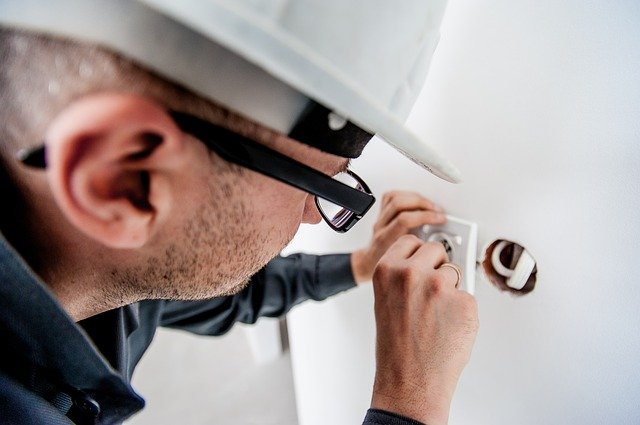# Practical Electricity – What’s tested for O Level PhysicsIn this post, let’s look at what’s tested in detail for practical electricity for O Level physics.

I go through the points in detail in my video here.

This chapter has many definitions. You can find a list of definitions for O Level Physics here. There are also some calculation questions from this chapter. For calculation questions, it’ll involve the use of formulae. You can find a compilation of the formulae for O Level Physics here.

Below is a checklist of what you need to know for O Level Physics on the topic practical electricity.

## Checklist for Practical Electricity for O Level Physics

✓ describe the use of the heating effect of electricity in appliances such as electric kettles, ovens and
heaters
✓ use P = VI and E = VI t in calculation questions

✓ calculate the cost of using electrical appliances using the energy unit of kW h
✓ compare the use of non-renewable and renewable energy sources in terms of energy conversion efficiency, cost per kW h produced and environmental impact

✓ state the hazards of damaged insulation, overheating of cables, and using electricity in damp conditions
✓ explain the use of fuses, circuit breakers, earthing of metal cases, and double insulation
✓ state the meaning of live, neutral and earth wire
✓ describe the wiring in a mains plug
✓ explain why switches, fuses, and circuit breakers are connected to the live conductor

Watch the video below to learn practical electricity in detail.

error: Content is protected !!